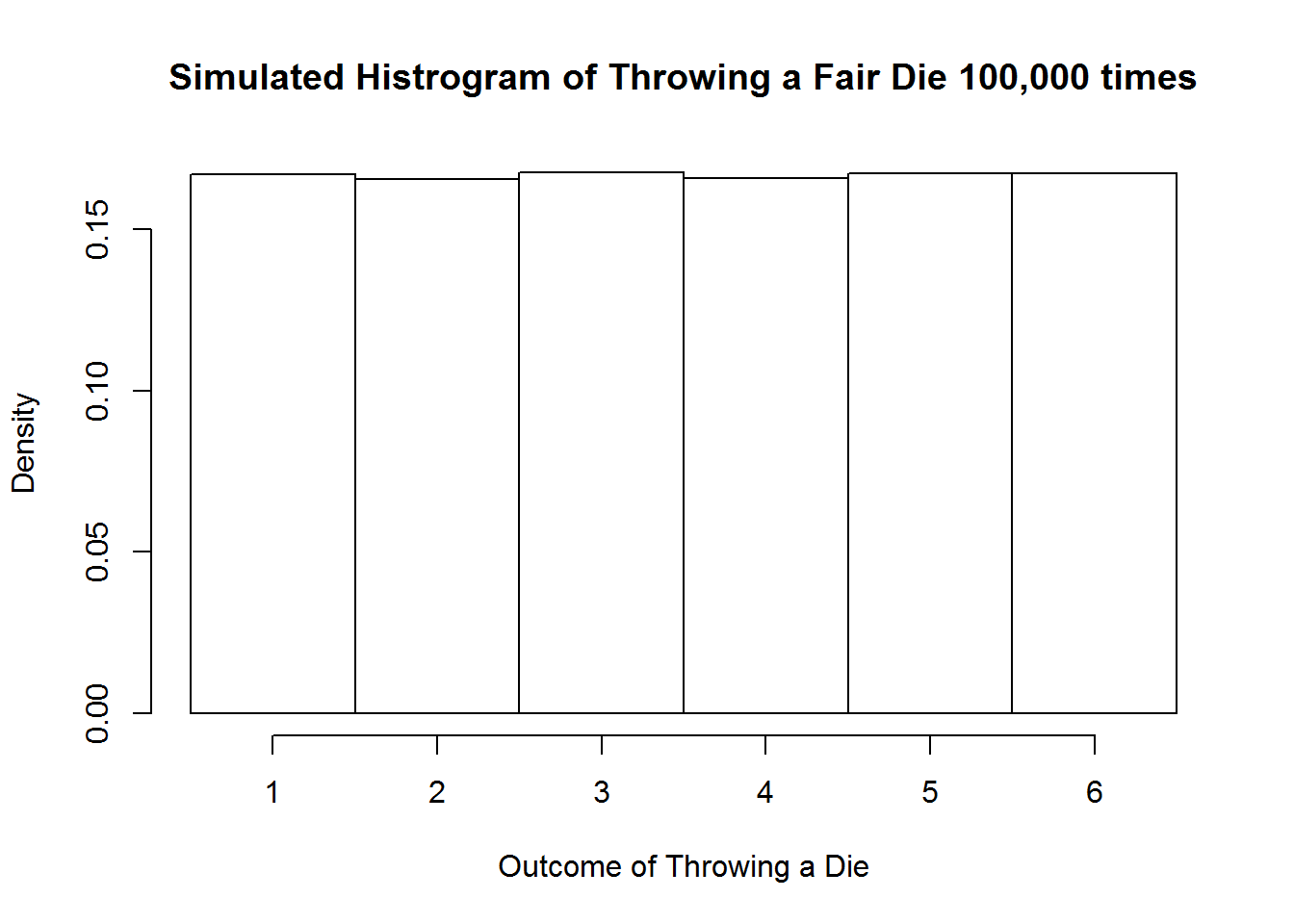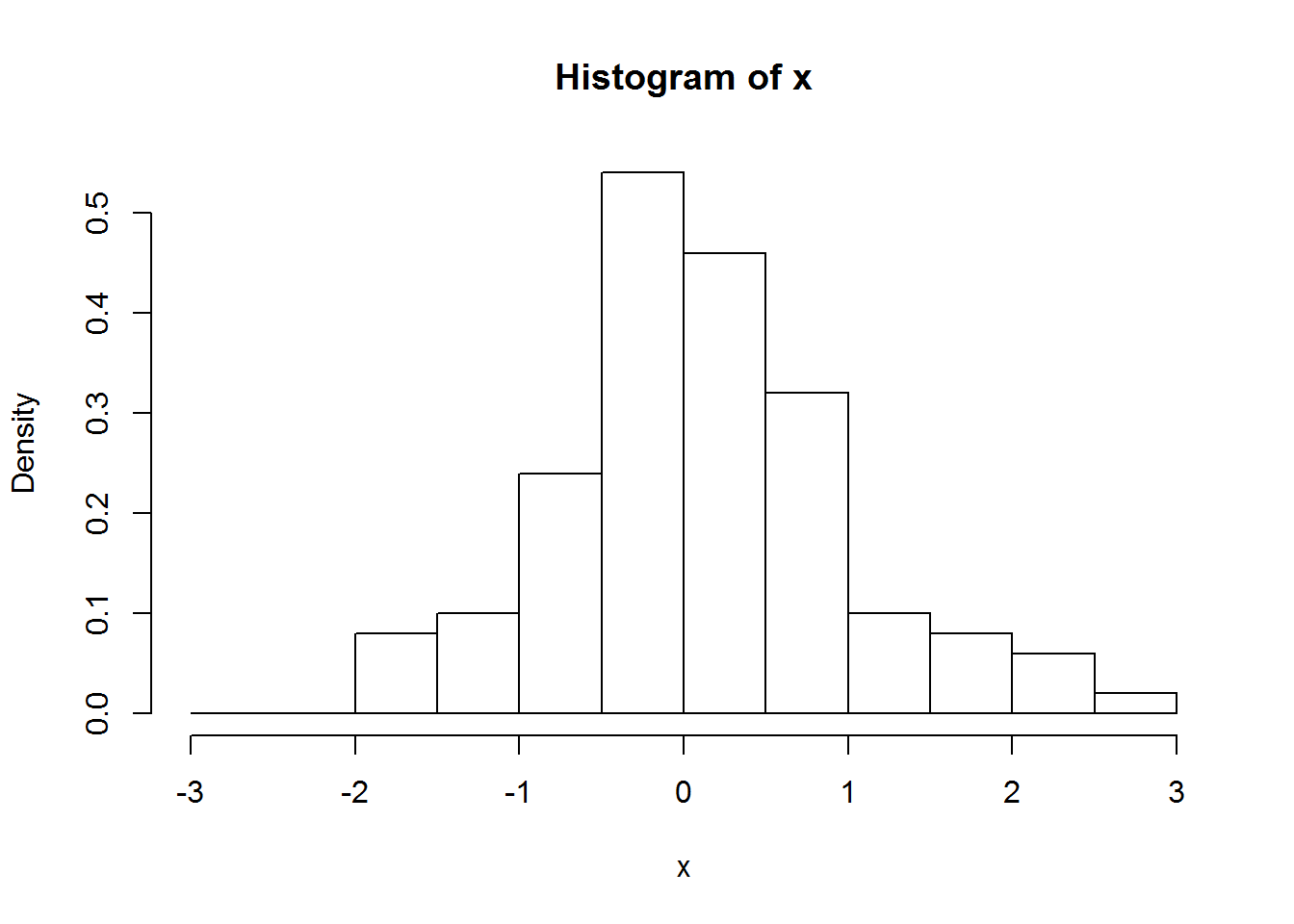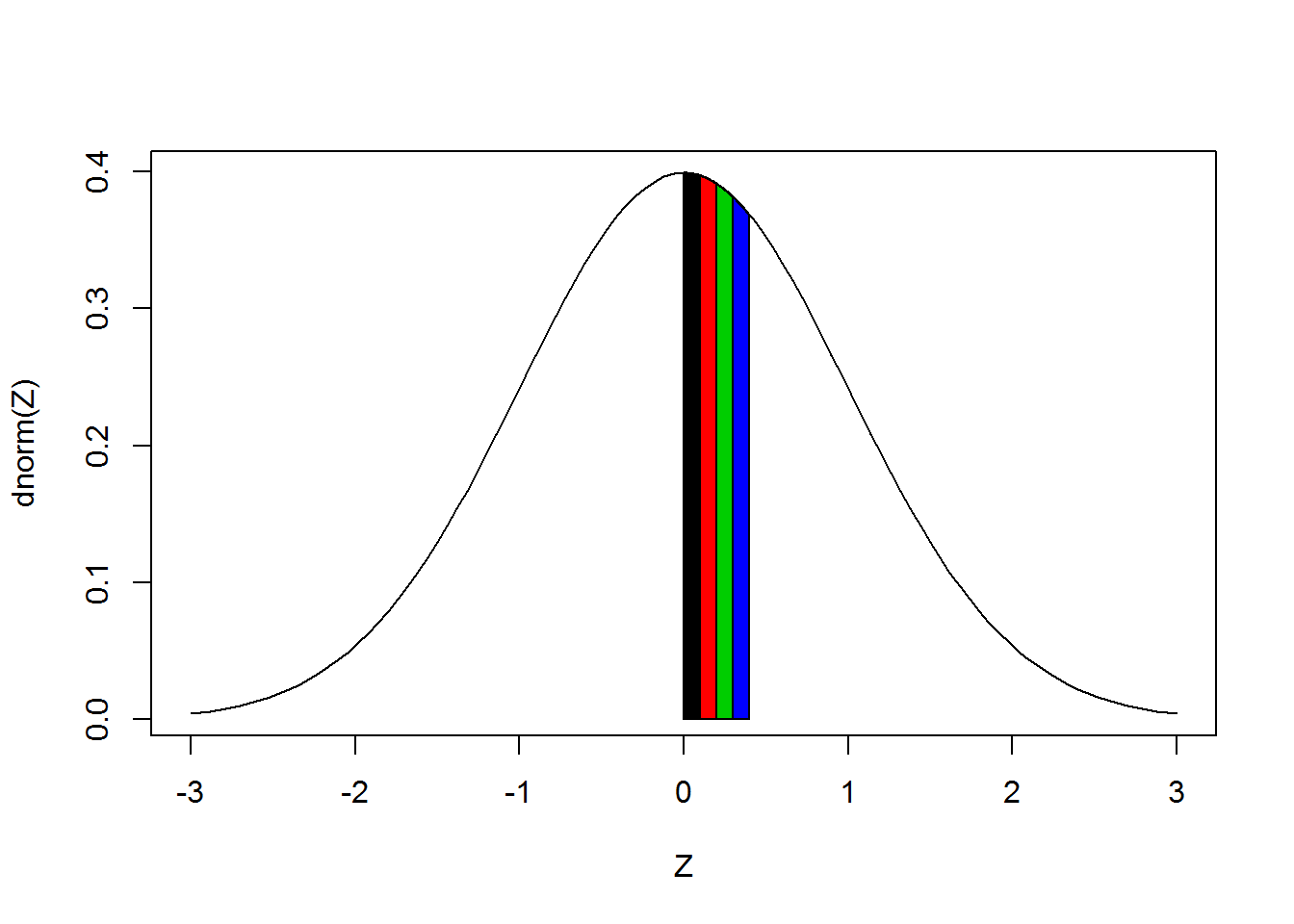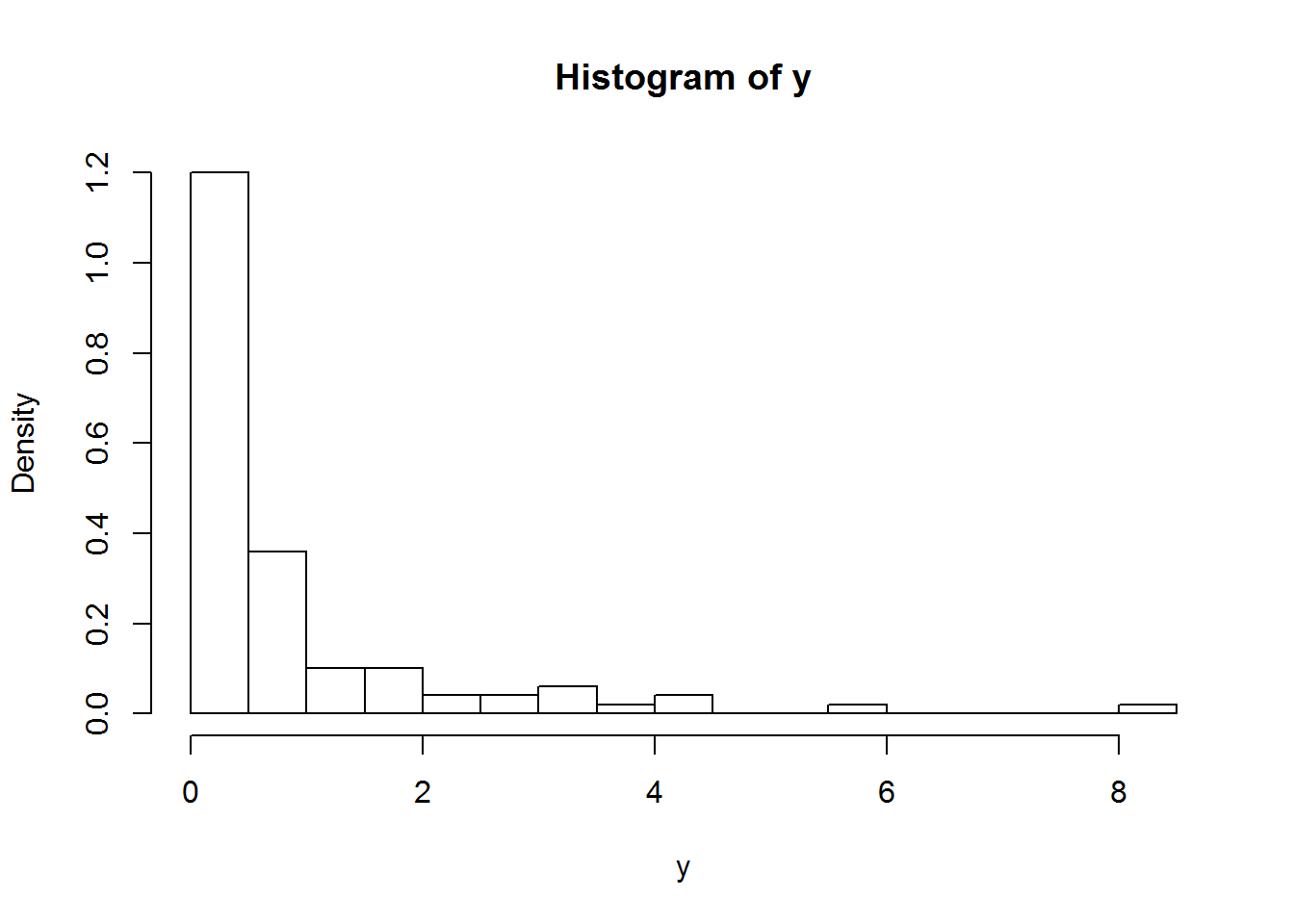Random Numbers and Pseudo Random Numbers

You probably have come across the fill-in-the-blank exercises similar to the following:

1. 1, 2, 4, 8, 16, 32, __
2. 1, 4, 9, 16, 25, __
3. 3, 4, 7, 11, 18, 29, __

Can you fill in the missing blanks? They are 64, 36, and 47. In the first sequence, each number is twice of the previous one. The second sequence is a square sequence: 12, 22, 32, 42, 52, 62. For the third sequence, the 3rd number and beyond are obtained by the sum of the previous two numbers: 7=4+3, 11=7+4, 18=11+7, 29=18+11, 29+18=47. The numbers in the above 3 sequences are not random, in the sense that they follow a certain rule. You can predict the numbers in the sequence by just observing a few of them.

By contrast, sequences of random numbers do not follow any pattern in the appearance of numbers. Take for example, the outcomes of throwing a die. If you throw a die 10 times, you may get a sequence such as 1, 6, 2, 1, 5, 6, 3, 3, 3, 6. You cannot predict what the next outcome will be based on the observed outcomes. Computers can generate numbers that appear to be random. For example, the commands

rnorm(10)
  0.93728663  1.64729779  1.76768042 -0.01816226 -0.86260006
  0.27750780 -0.86509918  0.02349651  1.09932461 -0.14221929

generates 10 numbers that appear to be random. It is not easy to predict what number you will get the next time you run rnorm() again. However, the seemingly random numbers generated by computers are not truly random. They are generated by somewhat complicated algorithms. If you figure out the algorithm, you will be able to predict what the next number will be. For example, I can tell you that the above 10 numbers are generated using R’s rnorm() function with a seed number 39135. The same 10 numbers can be reproduced by opening an R console, typing set.seed(39135) and then rnorm(10). To find out what the next number will be, just type

rnorm(1)
 0.4126871

For this reason, these numbers are called pseudo random numbers. Even though pseudo random numbers are not true random numbers, they are easy to generate and share many statistical properties of true random numbers. They are widely used in many applications to simulate random numbers. For simplicity, we do not distinguish random numbers and pseudo random numbers hereafter.

Random Number Distribution

By definition, the outcomes of random numbers cannot be predicted, but in many cases a particular sequence of random numbers satisfy well-defined statistical properties. For example, suppose you throw a die many times and record the outcomes. Even though you cannot predict the outcome of each throw, when you examine the outcomes you find that each of the number ‘1’, ‘2’, ‘3’, ‘4’, ‘5’, and ‘6’ occurs roughly equal number of times. We say that these random numbers follow a uniform distribution. The distribution can be visualized by a histogram:This histogram is generated using R’s random number generators to simulate throwing a fair die 100,000 times. We will talk about using R to do simulations later in the course. We see that the fraction of occurrence of each number is about 1/6, exactly what is expected for a fair die.

By contract, the random numbers generated by the rnorm() function do not look like that. Let’s examine 100 random numbers generated by the rnorm() function:

set.seed(389248467)
x <- rnorm(100)
x
 -0.33642489 -1.85866653  0.84962858  1.08095693  0.78111295
 -0.88880093  0.91231255 -0.90352990 -1.69217365 -1.88810130
 -1.24397742  0.48399114  0.63844970 -1.32033456  0.88547441
  2.07812710  0.01747082 -0.09377385  0.40994825 -0.61752212
  1.82240128 -1.15521149  0.90239205  2.38523344  0.87707807
  0.67067971 -0.33708050  1.77617463  0.26243972 -0.48282886
  0.78471407 -0.67953414 -0.15899528  1.02022475 -0.62977814
 -0.50065470 -0.21610114 -0.19481166  0.84457164  0.22253655
 -0.35023048 -0.48023591  0.03475135  0.01375636  0.13610260
  0.80172257 -0.71740883 -0.15670132 -0.14159004  1.57662435
 -0.03143710  0.61773241  1.35306081  0.27539103  0.47239784
 -0.35082932  1.69875570 -0.43854974 -0.07726839 -0.23165693
 -0.39260988  2.83296608  0.45853024 -0.26649941  0.26208313
 -0.96151444 -0.42345906  1.31624364  1.04636080 -1.53503436
  0.12380020 -1.13593939 -0.17435843 -0.52715083 -0.81702084
 -0.79645346 -0.06387693 -0.95573420 -0.23334426 -0.15968870
  0.25665795 -1.28189767  0.25019336  0.08593316  0.39537421
 -0.43674779  0.65546434  2.03991422  0.88433928 -0.04689556
  0.76062998 -0.14072072 -0.09427394  0.47887879  0.25473042
  0.66439995  0.20484101  0.39436547  0.47743103  0.12530810

These numbers are not uniformly distributed. For example, only 4 numbers are larger than 2. To see the distribution, we plot the histogram:This shows that most of the numbers are in the range (-1,1). The farther away it is from 0, the less fraction of numbers we tend to see. The distribution follows roughly the standard normal curve. This is because the rnorm() function simulates random numbers drawn from the standard normal distribution (mean=0 and standard deviation=1). You can think of it as follows. Suppose there is a box that contains trillions of tickets, each ticket has a number written on it. The numbers in the box follows the standard normal distribution. That is to say, the fraction of the tickets with numbers between $$Z_1$$ and $$Z_2$$ is given by the area under the normal curve between $$Z=Z_1$$ and $$Z=Z_2$$. For example, the fraction of tickets with numbers between $$Z=0$$ and $$Z=0.1$$ is given by the area of the shaded black region in the plot below; the fraction of the tickets with numbers between $$Z=0.1$$ and $$Z=0.2$$ is given by the area of the shaded red region; the fraction between 0.2 and 0.3 is given by the area of the shaded green region; the fraction between 0.3 and 0.4 is given by the area of the shaded blue region; and so on.Recall that the total area under the standard normal curve (actually for all probability density curves) is 1, meaning that all of the numbers in the box are between $$-\infty$$ and $$\infty$$. However, since the curve falls rapidly as we move away from $$Z=0$$, the area under the curve beyond large values of $$|Z|$$ are small, meaning that the fractions of tickets with large values of $$|Z|$$ is small.

Imagine you randomly draw tickets from this box. Since the number of tickets is large, it doesn’t really matter if you draw them with or without replacement. Since there are more tickets in the central region, the majority of the tickets you draw will have numbers in the central region but ocassionally you will get larger values of |Z|. The rnorm() function simulates the random draws from this hypothetical “standard normal box”.

Random Numbers From Transforming and Combining Other Random Numbers

Random numbers can also be created from existing random numbers. For example, a new set of random numbers can be generated from the random numbers stored in x above:

y <- x^2
y
 0.1131817093 3.4546412682 0.7218687258 1.1684678865 0.6101374361
 0.7899670883 0.8323141885 0.8163662762 2.8634516635 3.5649265004
 1.5474798203 0.2342474219 0.4076180142 1.7432833389 0.7840649224
 4.3186122483 0.0003052296 0.0087935354 0.1680575651 0.3813335653
 3.3211464162 1.3345135906 0.8143114137 5.6893385662 0.7692659413
 0.4498112775 0.1136232666 3.1547963130 0.0688746049 0.2331237125
 0.6157761736 0.4617666439 0.0252794991 1.0408585341 0.3966205107
 0.2506551273 0.0466997013 0.0379515810 0.7133012593 0.0495225169
 0.1226613900 0.2306265253 0.0012076564 0.0001892375 0.0185239170
 0.6427590827 0.5146754326 0.0245553052 0.0200477389 2.4857443382
 0.0009882914 0.3815933264 1.8307735676 0.0758402180 0.2231597170
 0.1230812120 2.8857709338 0.1923258756 0.0059704042 0.0536649313
 0.1541425159 8.0256967851 0.2102499849 0.0710219336 0.0686875675
 0.9245100132 0.1793175769 1.7324973084 1.0948709147 2.3563304871
 0.0153264907 1.2903582874 0.0304008607 0.2778879973 0.6675230572
 0.6343381085 0.0040802627 0.9134278642 0.0544495427 0.0255004810
 0.0658733032 1.6432616311 0.0625967178 0.0073845073 0.1563207663
 0.1907486345 0.4296334970 4.1612500073 0.7820559647 0.0021991932
 0.5785579730 0.0198023202 0.0088875753 0.2293248927 0.0648875882
 0.4414272966 0.0419598398 0.1555241217 0.2279403887 0.0157021189

Even though the new 100 numbers are related to the random numbers in x, they are still random numbers: you cannot predict what the next number will be based on the 100 numbers shown here. However, the statistical property of this new set of random numbers is different from that of x. For example, there are no negative numbers in the new set. The histogram of y is very different from x:While x follows the standard normal distribution, y follows another distribution. The distribution of y can be calculated theoretically. It is called the $$\chi^2$$ distribution with one degree of freedom. You can think of doing the experiment of drawing 100 numbers from the hypothetical “standard normal box”. Instead of recording the numbers on the tickets, you record the square of the numbers on the tickets.

Many other sets of random numbers can be created by transforming the random numbers in x. For example, z1 <- x^3, z2<- 1/x, z3 <- sin(x), … etc. In each case, the new set of random numbers follow a different distribution. In addition to transformation of one set of random numbers, we can also create a new set of random numbers by combining different sets of random numbers. For example, suppose we draw 100 random numbers from a hypothetical “standard normal box”, then draw another 100 random numbers, and then draw another 100 numbers. We can simulate this experiment using the following R commands:

set.seed(764856)
x1 <- rnorm(100)
x2 <- rnorm(100)
x3 <- rnorm(100)

To see the outcomes of this experiment, we type

x1
 -1.56989914  0.27737857 -0.17448396 -0.15567664  1.05962871
 -1.30988373 -0.54054191 -0.17871592  0.79431777  1.77040310
  0.24786164 -1.11680260  0.50618959  1.50116189 -0.09661000
 -1.09896811  0.89777029  0.21967476  1.02347927  1.50027705
  0.76815818  0.41960381 -1.26471720  0.23228270  0.84444443
  0.30149477 -0.29571293  1.26140638 -0.57037786  0.37728694
 -0.61342098 -0.41655880 -0.25793311  0.90775372  0.17367510
 -0.77158407 -1.40522480 -0.75774245 -1.41014449  1.13393549
  0.32000688  1.91108332 -1.02753867 -1.42282033  0.35538182
  1.30721971 -0.37686105 -0.16501434 -1.74558569  1.10943605
 -0.21237578 -0.84149854  0.08517314 -0.53607313 -1.08238906
 -1.61640959  0.62726836 -0.04077411  0.07548332 -1.18251193
  1.07561579 -1.13829291 -1.66276646 -0.90579044  1.46359882
  0.42014460  1.82138351  0.25628093 -0.82311590  0.48546065
 -1.99027056 -1.35885056 -1.29179601  0.66563488 -1.50052117
  0.83122896  1.51476641 -0.38744094  0.82813844 -0.35984275
  0.14356890 -0.94315147 -0.43423079 -0.86548613  0.70510493
 -0.35946978  0.05988018 -1.09657945 -1.62081744 -0.33952879
  0.16541282  0.48557704 -1.44905109  1.51352739  0.15912290
  0.67586570 -0.62062810 -0.34801994 -1.19256226 -0.98994779
x2
 -0.860265973  0.290678524 -0.073283987 -0.212095502 -0.759340538
  0.141960335  0.465306911  0.173300238  0.371368467  0.658264376
 -2.076644459 -1.292762500 -1.374796345  0.308679481 -1.006216879
  1.412313214 -1.070822011  0.339852753  0.439338551 -0.015646754
 -0.287268700 -0.702062330  0.641910124  0.521238324 -0.232395006
 -0.107703830 -1.167782047 -0.801714774 -1.456864719 -2.007132245
 -0.742263865 -0.985709733  0.608655830 -1.341735992  0.380823828
 -1.325384935  0.599831860 -1.171720853 -1.143084279 -0.338415016
  0.067126713 -0.372103257  0.388680874  1.644737187 -2.025779295
  0.976733062 -0.316468612  0.475272515 -1.323069322 -0.478743455
 -0.423173923 -0.263237056 -0.784601478 -0.544535135 -0.732538509
 -1.917649346 -0.926345515 -0.234192685 -0.100036322 -2.354518176
  0.106833494 -1.189891370 -0.626555705  1.641385266 -1.476179949
  0.550559049  1.019246462  1.513995085  0.424183021 -1.350528286
 -0.165525583 -0.120803080 -1.732234387  0.952274855 -1.716873841
  0.665643107 -0.479247747 -3.033001554 -0.351822362 -1.521936462
 -1.286285400  0.604810775  0.100728191 -0.551672697 -0.613001248
 -0.542721594 -1.338629655 -0.213902046  0.005469832 -0.894096579
  2.745007028  0.520232451 -0.357146688  2.849780208  1.670955483
  0.815042288  0.093814633 -0.180128307 -0.679176114 -0.795664836
x3
  0.789491656 -0.428773857 -0.004451979  1.269232645 -0.051647781
 -0.066589858 -0.648634245  0.276969771  0.140654010 -0.808308276
 -0.076436932  0.511148633  0.090902723  0.517748567 -0.354745177
 -0.862677961 -0.378356437  0.753806862  0.765405924 -0.967240447
 -0.160775629 -0.951106158  0.085793013  0.352847115  1.835602353
 -0.939500700  0.321279933 -0.388913507  1.164002359 -1.450849226
  1.385467049  0.191128627 -1.901605458 -0.308854682 -0.933424121
 -0.152954207  0.236287565  0.617729229 -0.322480842 -1.458479413
 -0.990432157 -1.413272946  0.594344711 -0.174667832  0.691274091
 -0.493905427 -0.426066260 -0.484788912  0.069343429  1.118955902
 -0.079358000  0.430337772 -0.125986391 -0.489180753  0.522330403
  1.965114792 -0.234914065 -0.055431792 -0.978963620 -0.051563889
  1.127673928  0.486589000 -1.286946871  0.040664296  0.651547534
  0.407362665 -1.451991144 -0.002698506  1.679512712  0.204938286
 -0.257614929 -0.606784910 -0.459843793  1.027801732  0.043451202
 -0.835943800 -0.005825615 -0.698470685  0.287640810 -0.727696793
  0.731907208 -0.574634905 -0.562905971 -0.925444672  0.278726517
  0.476111365  0.260630354 -0.176800552 -0.316708585  0.031575627
 -0.004682573 -0.948204414  1.827630136  1.572350142 -1.150703713
 -1.221416396 -1.170852083  0.489432540  1.678925656  0.952789026

We can combine them into a new set of random numbers in the following way. Take the first element of x1, x2 and x3: -1.5698991, -0.860266 and 0.7894917. Square them, take the sum and write down the result as the first random number in our new set:
(-1.5698991)2 + (-0.860266)2 + (0.7894917)2 = 3.8279379 <— first random number in our new set.

Do the same operation for the other 99 elements. In R, this can be done by the command

y <- x1^2 + x2^2 + x3^2
y
  3.82793794  0.34527990  0.03583501  1.68017122  1.70207856
  1.74038232  0.92942246  0.13868461  0.78863881  4.22100139
  4.37973021  3.17975585  2.15455620  2.61683361  1.14765004
  3.94657279  2.09580487  0.73198168  1.82637441  3.18663013
  0.69843910  1.57356179  2.01891864  0.45014573  4.13652984
  0.98516077  1.55438184  2.38514635  3.80268720  6.27588876
  2.84675989  1.18167506  4.05309473  2.71966350  1.04647042
  2.37538219  2.39028680  2.32869278  3.39914306  3.52749663
  1.08786626  5.78804071  1.56015417  4.76008697  4.70793786
  2.90677343  0.42370909  0.48813398  4.80239034  2.71210595
  0.23047733  0.96260414  0.63872651  0.82319072  1.98100779
 10.15183511  1.30676623  0.05958143  0.97407477  6.94474915
  2.44001120  2.94832106  4.81359660  3.51625550  4.74574294
  0.64558109  6.46457954  2.35786831  3.67821397  2.10159840
  4.05494109  2.22925617  4.88082923  2.40627359  5.20110758
  1.83282436  2.52422961  9.83707021  0.89232948  2.97532002
  2.21083032  1.58553604  0.51556568  1.90985684  0.95063197
  0.65044728  1.86344317  1.27949902  2.72738343  0.91568551
  7.56244691  1.40551847  5.56753474 12.88429736  4.14153136
  2.61294639  1.76487502  0.39310830  4.70227629  2.52088609

We have just generated a new set of 100 random numbers and stored them in the variable y. We can also do it directly using the following commands:

set.seed(764856)
y2 <- rnorm(100)^2 + rnorm(100)^2 + rnorm(100)^2

We can verify that y and y2 are the same:

y-y2
 0 0 0 0 0 0 0 0 0 0 0 0 0 0 0 0 0 0 0 0 0 0 0 0 0 0 0 0 0 0 0 0 0 0 0
 0 0 0 0 0 0 0 0 0 0 0 0 0 0 0 0 0 0 0 0 0 0 0 0 0 0 0 0 0 0 0 0 0 0 0
 0 0 0 0 0 0 0 0 0 0 0 0 0 0 0 0 0 0 0 0 0 0 0 0 0 0 0 0 0 0

The new set of random numbers y follow a different distribution than any of x1, x2, and x3. It is also different from the distribution of x1^2, x2^2 and x3^2. The resulting distribution can be calculated theoretically. They are called the $$\chi^2$$ distribution with 3 degrees of freedom.

In Statistics, we encounter random numbers following different distributions. Some well-known distributions, e.g. $$\chi^2$$, t and F, in statistics arise from transforming and combining different sets of random numbers following a particular distribution.